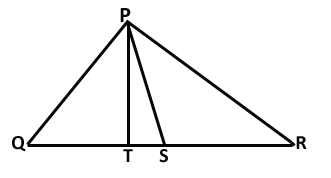# Class 9 Lines and Angles Important Questions

Lines and Angles Important Questions

1. The diagonals of the rectangle ABCD intersect at O. If ∠COD = 78°, then ∠OAB is:
1. 35o
2. 51o
3. 70o
4. 110o
2. If AB = x + 3, BC = 2x and AC = 4x – 5, then for what value of ‘x’, B lies on AC?
1. 2
2. 3
3. 5
4. 8
3. In the given Figure , POQ is a line. Ray OR is perpendicular to line PQ. OS is another ray lying between rays OP and OR. Prove that ∠ROS = 1/2(∠ QOS – ∠ POS).4. In the given Figure, if PQ || RS, ∠ MXQ = 135° and ∠ MYR = 40°, find ∠XMY.5. Prove that the angle formed by the bisector of interior angle A and the bisector of exterior angle B of a triangle ABC is half of angle C.
6. In the given Figure, the sides AB and AC of ∆ABC are produced to points E and D respectively. If bisectors BO and CO of ∠ CBE and ∠ BCD respectively meet at point O, then prove that ∠BOC = 90° – 1/2 ∠BAC.7. Bisectors of interior ∠B and exterior ∠ACD of a Δ ABC intersect at the point T.Prove that ∠ BTC = ½ ∠ BAC.
8. A transversal intersects two parallel lines. Prove that the bisectors of any pair of corresponding angles so formed are parallel.
9. In the figure, PS is the bisector of ∠QPR and PT ⊥ QR. Show that ∠TPS = ½(∠Q – ∠R).10. If two parallel lines are intersected by a transversal, prove that the bisectors of the two pairs of interior angles enclose a rectangle.
11. The angles of a triangle are arranged in ascending order of magnitude. If the difference between two consecutive angles is 10°, find all the three angles.
12. The exterior angles obtained on producing the base of a triangle both ways are 100° and 120°. Find all the angles of the triangle.